# What is freefall acceleration

## Free fall (physics)

Free fall is covered in this article. We explain what is meant by free fall and how to calculate it. This article belongs to the field of physics / mechanics.A free fall is understood to mean the fall of a body close to the earth, without taking air resistance into account. In this case, the formulas for uniformly accelerated movement apply. We will go into exactly these again in the following section and provide corresponding examples.

Show:

### The free fall: formula

As already mentioned in the introduction, the formulas of the uniformly accelerated movement are used to calculate the free fall. These formulas are based on the assumption that an object is released from a certain height above the earth and the body "flies" directly towards the earth's surface.

Formula 1: free fall track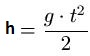Where:

• "h" is the fall distance in meters
• "g" is the acceleration due to gravity in meters per second square
• "t" is the fall time in seconds

An initial route is not taken into account here.

Note: The acceleration due to gravity is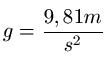Example:

An object falls towards the ground near the earth. How far does the body fall within 10 seconds?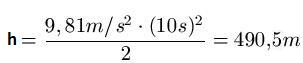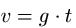Where:

• "v" is the speed of fall in meters per second
• "g" is the acceleration due to gravity in meters per second square
• "t" is the fall time in seconds

Note: An initial speed is not taken into account here.

Example 2:

An object falls in free fall for 8 seconds. How fast is the object then?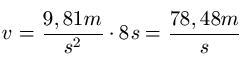Left:

### Who's Online

We have 231 guests online